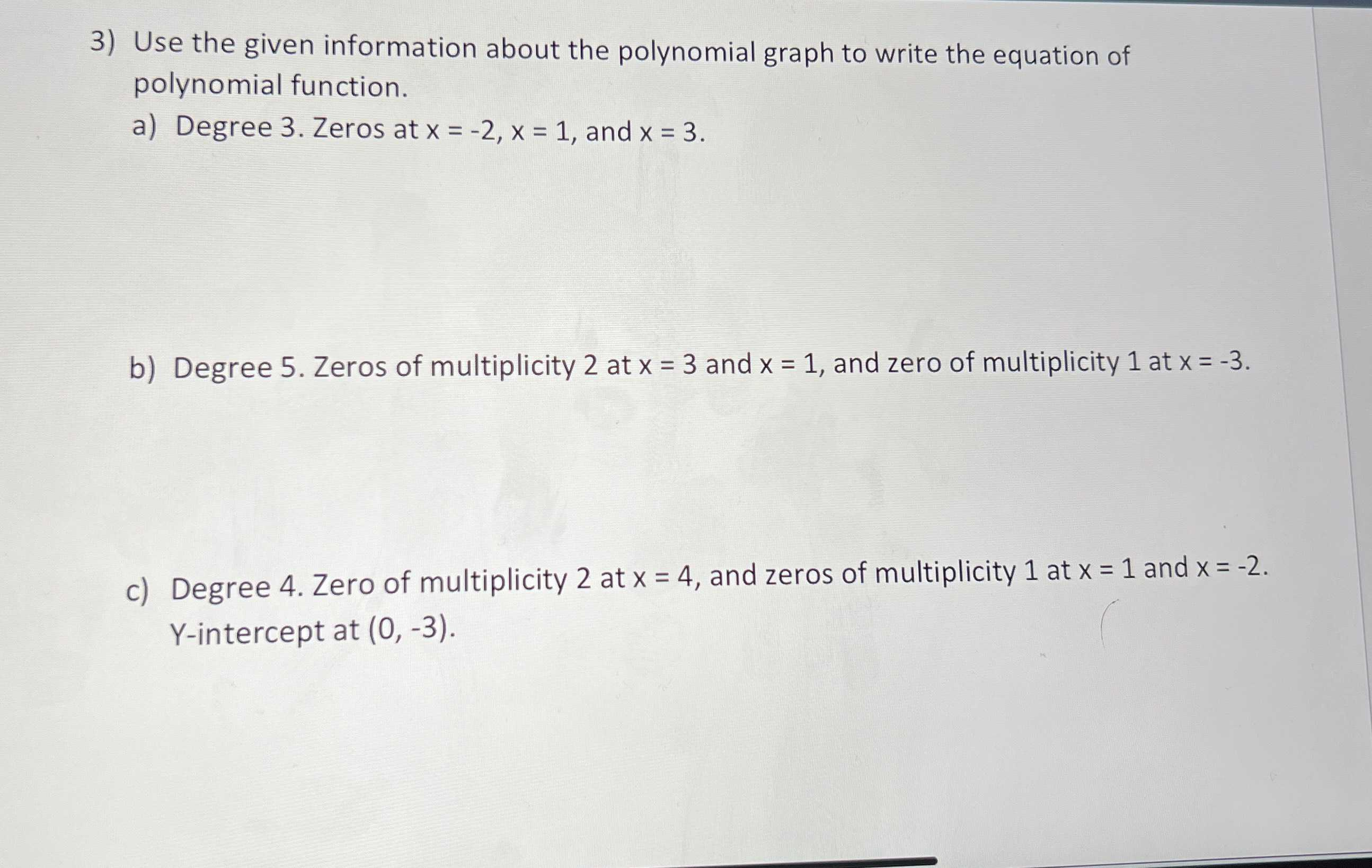### Still have math questions?

Algebra
QuestionUse the given information about the polynomial graph to write the equation of polynomial function.

a) Degree $$3$$ . Zeros at $$x = - 2 , x = 1$$ , and $$x = 3$$ .

b) Degree $$5$$ . Zeros of multiplicity $$2$$ at $$x = 3$$ and $$x = 1$$ , and zero of multiplicity $$1$$ at $$x = - 3$$ .

c) Degree $$4 .$$ Zero of multiplicity $$2$$ at $$x = 4$$ , and zeros of multiplicity $$1$$ at $$x = 1$$ and $$x = - 2$$ . Y-intercept at $$( 0 , - 3 )$$ .